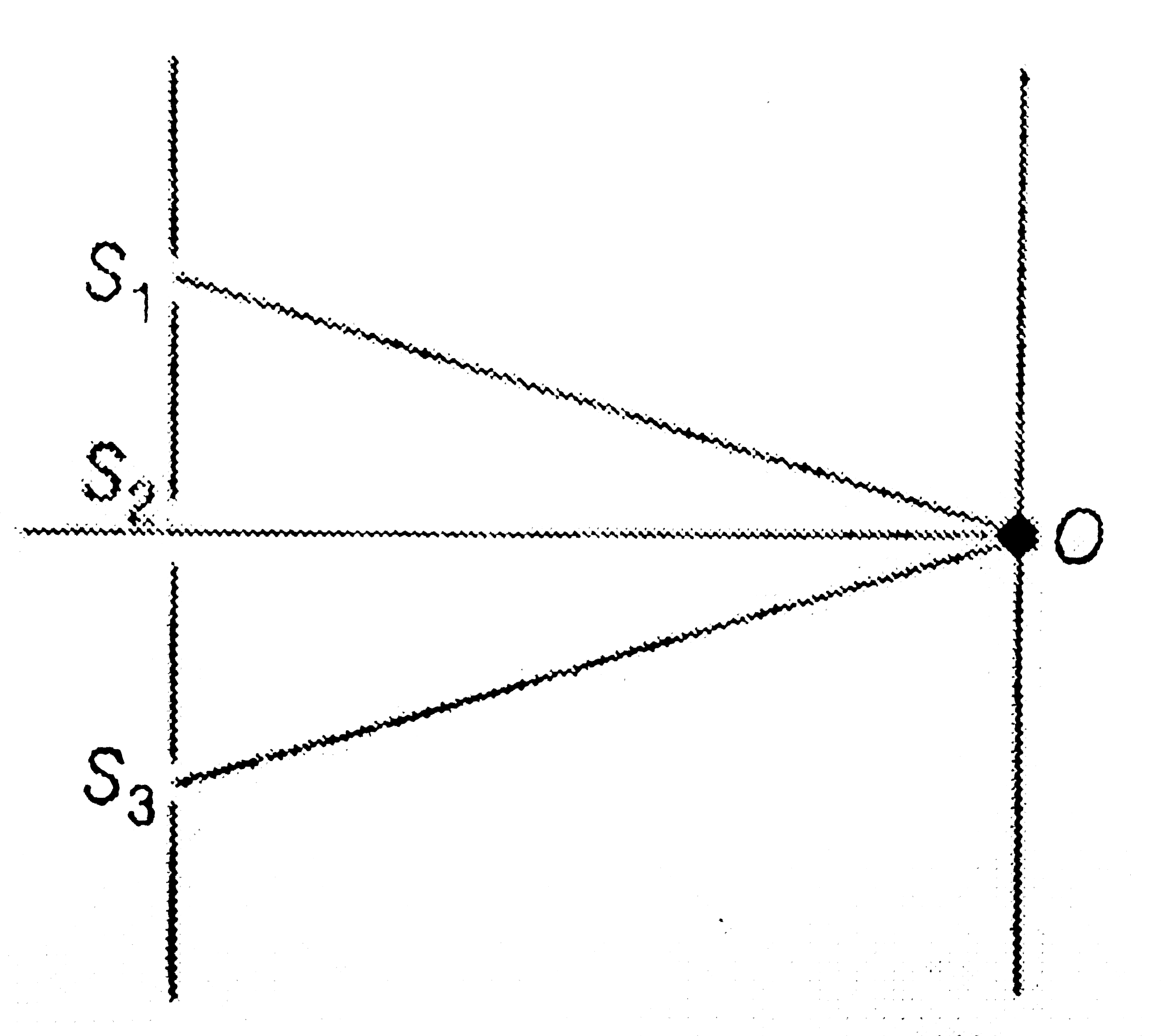# In the figure shown S_(1)O-S_(2)O=S_(3)O-S_(2)O=(lambda)/(4), Intensity at O due to any one of the slits is I_(0). What is the intensity due to al

18 views
in Physics
In the figure shown S_(1)O-S_(2)O=S_(3)O-S_(2)O=(lambda)/(4), Intensity at O due to any one of the slits is I_(0). What is the intensity due to all the three coherent sources S_(1), S_(2) and S_(3) ?A. 3I_(0)
B. I_(0)
C. 5I_(0)
D. 9I_(0)

by (73.0k points)